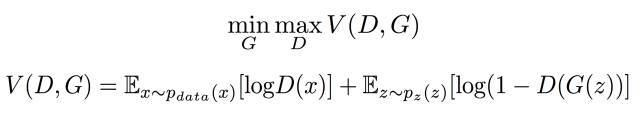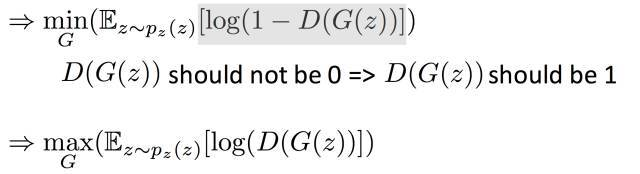D的目的是增大目标函数V，所以在实际训练过程中我们只需要在V前面整体加上一个负号就行，这样最大化就可以转化为最小V一般的优化器都是最小化损失 函数所以在操作上我们要将最大改为最小。这样我们就可以实现判别器D的损失函数的设计了。再看看G的损失函数，我们观察目标函数，第一项中是不含G的 所以我们可以不考虑第一项，仅仅对第二项讨论。最小化第二项就相当于下面操作：""" Loss Function """
# output of D for real images, D_real((64,1),介于(0,1)),D_real_logits未经历
# 过sigmoid，_临时存储net(64,1024)
D_real, D_real_logits, _ = self.discriminator(self.inputs, is_training=True, reuse=False)

# output of D for fake images G为由噪声z（64,62）生成的图片数据(64,28,28,1)
G = self.generator(self.z, is_training=True, reuse=False)

# D_fake((64,1),介于(0,1)),D_fake_logits未经历过sigmoid，_临时存储net(64,1024),
# 送入鉴别器的是G生成的假的数据
D_fake, D_fake_logits, _ = self.discriminator(G, is_training=True, reuse=True)

# get loss for discriminator
d_loss_real = tf.reduce_mean(
tf.nn.sigmoid_cross_entropy_with_logits(logits=D_real_logits,
labels=tf.ones_like(D_real)))
d_loss_fake = tf.reduce_mean(
tf.nn.sigmoid_cross_entropy_with_logits(logits=D_fake_logits,
labels=tf.zeros_like(D_fake)))
#d_loss为生成器和鉴别器传出的loss之和
self.d_loss = d_loss_real + d_loss_fake

# get loss for generator
#g_loss=-log(sigmoid(D_fake_logits))等价于g_loss=-log(D(G(z))
self.g_loss = tf.reduce_mean(
tf.nn.sigmoid_cross_entropy_with_logits(logits=D_fake_logits,
labels=tf.ones_like(D_fake)))

""" Training """
# divide trainable variables into a group for D and a group for G
t_vars = tf.trainable_variables()
d_vars = [var for var in t_vars if 'd_' in var.name]
g_vars = [var for var in t_vars if 'g_' in var.name]

with tf.control_dependencies(tf.get_collection(tf.GraphKeys.UPDATE_OPS)):
.minimize(self.d_loss, var_list=d_vars)
.minimize(self.g_loss, var_list=g_vars)


tensorflow-GANs

pytorch-GANs